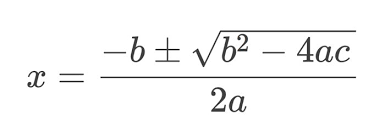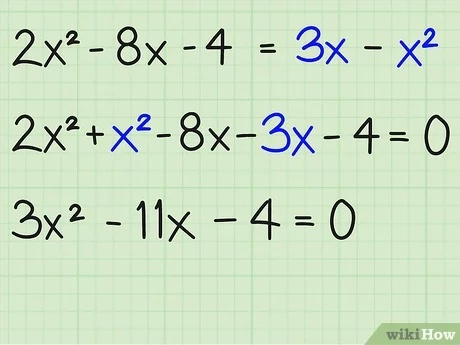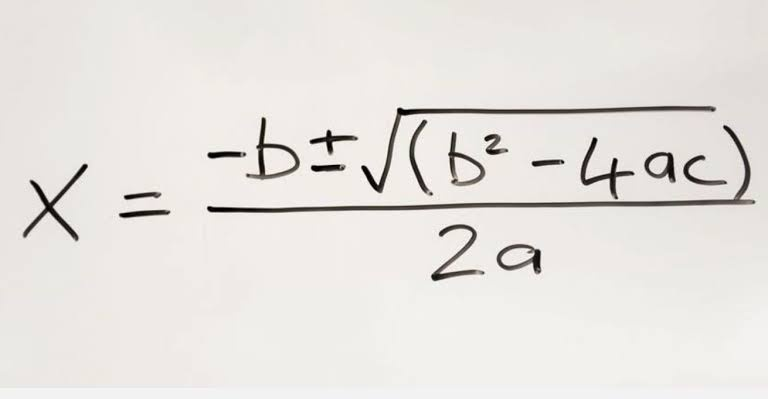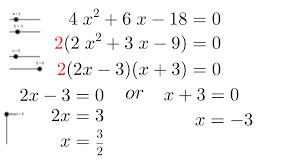Education

# Almighty Formula: Solve Any Quadratic Equation Using the Almighty Formula

## How To Solve Any Quadratic Equation Using The Almighty Formula

Adding one to another one is how mathematics started and as the years passed, man burning desire to know the unknown became stronger; this made mathematics to be broad.The almighty formula is a formula used to solve quadratic equation. Solving quadratic equations comes in three different methods. You can solve by factorization method, completing the square method, and the almighty formula method. Many of us are used to the factorization method where the coefficients of x² is used to multiply the constant. See example below,2x²+3x -5=0

Using the factorization method

2x²+3x-5=0

2x²-2x+5x-5=0

2x(x-1)+5(x-1)

(2x+5)(x-1)=0

2x+5=0 or x-1=0

2x = -5 or x = 1

x = -5/2 or x = 1

The almighty formula is,The above formula is used to solve quadratic equations you can practice my using it to solve the example below.2x²-x+3=0

It will be simpler if you substitute the followings in the equation

a=2, b= -1, C= 3

## How To Solve Any Quadratic Equation Using The Almighty Formula

There are four different methods that can be used to solve a quadratic equation. Which are:

1. Factorization;
2. Completing the square;
4. Graphical solution.
Each of the four methods above can be used to solve specific types of quadratic equation, however our main focus shall be to apply the general quadratic formula also known as the “Almighty formula”.
As the alias implies, it can be used to solve any standard quadratic equation. This formula works where all else have failed.
For a standard quadratic equation of the form: ax2 + bx + c = 0;

`EXAMPLE: Solve 4x2-11x - 21 = 0`

There are equations that can’t be reduced using the above two methods. For such equations, a more powerful method is required. A method that will work for every quadratic equation. This is the general quadratic equation formula. We define it as follows: If ax2 + bx + c = 0 is a quadratic equation, then the value of x is given by the following formula:

Just plug in the values of a, b and c, and do the calculations. The quantity in the square root is called the discriminant or D. The below image illustrates the best use of a quadratic equation.

Example 3: Solve: x2 + 2x + 1 = 0

Solution: Given that a=1, b=2, c=1, and
Discriminant = b2 − 4ac = 22 − 4×1×1 = 0
Using the quadratic formula,  x = (−2 ± √0)/2 = −2/2
Therefore, x = − 1

## More Solved Examples For You

Question 4: Find the value of x:  27x− 12 = 0
A) 2/3    B) ± 2/3     C) Ambiguous    D) None of these

Answer : B) Here, a =  27, b = 0 and c = -12. Hence, from the quadratic formula, we have:
x = − 0 ± √02 – 4(27)(-12)/2 (27)
Thus x = ± √(4/9) = ± 2/3

Question 5: What is the formula for solving quadratic equation?

Answer: The general quadratic equation formula is “ax2 + bx + c”. In this formula, a, b, and c are number; they are the numerical coefficient of the quadratic equation and ‘a’ is not zero a  0.

Question 6: What is quadratic equation?

Answer: Simply, a quadratic equation is an equation of degree 2, mean that the highest exponent of this function is 2. Moreover, the standard quadratic equation is ax2 + bx + c, where a, b, and c are just numbers and ‘a’ cannot be 0. An example of quadratic equation is 3x2 + 2x + 1.

Question 7: Name of different method by which you can solve quadratic equation?

Answer: There are various methods by which you can solve a quadratic equation such as: factorization, completing the square, quadratic formula, and graphing. These are the four general methods by which we can solve a quadratic equation.

Question 8: What are the three form of quadratic function?

Answer: The three function are listed below which can be written as:

1. Standard Form: y = ax2 + bx + c, here a, b, and c are just numbers.
2. Factored Form: y = (ax + c) (bx + d) here also a, b, and c are just numbers.

Vertex Form: y = a (x + b)2 + c, and here also a, b, and c are numbers.